Refer to our Texas Go Math Grade 4 Answer Key Pdf to score good marks in the exams. Test yourself by practicing the problems from Texas Go Math Grade 4 Lesson 5.6 Answer Key Add and Subtract Mixed Numbers.

Essential Question

How con you add and subtract mixed numbers with like denominators?
Answer: First convert the mixed fraction to the fraction
if the denominators are same then you can directly add or subtract the numerators.

Unlock the Problem

After a party, there were 1$$\frac{4}{6}$$ quesadillas left on one tray and 2$$\frac{3}{6}$$ quesadillas left on another tray. How much otthe quesadillas were left?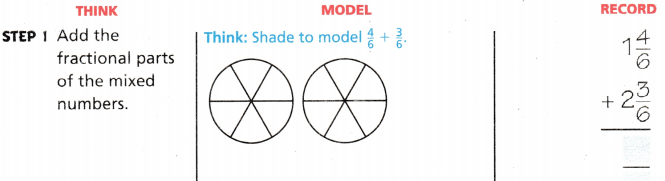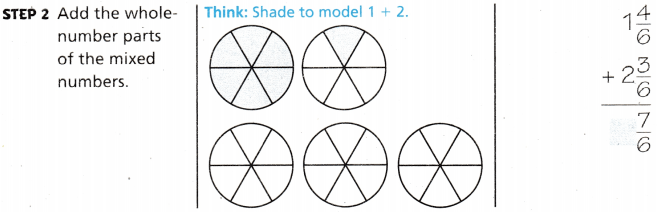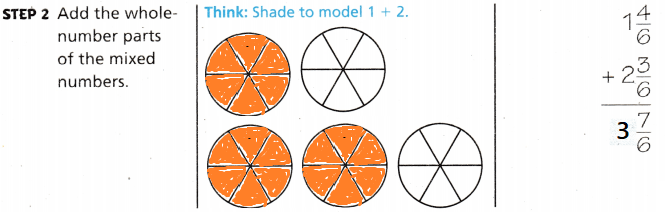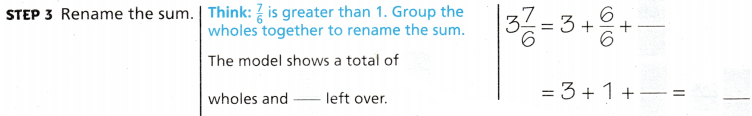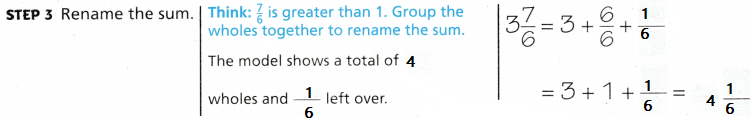So, 4$$\frac{1}{6}$$  quesadillas were left.

Math Talk

Mathematical Processes
When modeling sums such as $$\frac{4}{6}$$ and $$\frac{3}{6}$$, why is it helpful to combine parts into wholes when possible? Explain.
If we make a whole then adding the remaining fractions will be easy.

Example Subtract mixed numbers.

Alejandro had 3$$\frac{4}{6}$$ quesadillas. His family ate 2$$\frac{3}{6}$$ of the quesadillas. How many quesadillas are left?

Find 3$$\frac{4}{6}$$ – 2$$\frac{3}{6}$$

Model

Shade the model to show 3$$\frac{4}{6}$$.
Then cross out 2$$\frac{3}{6}$$ to model the subtraction.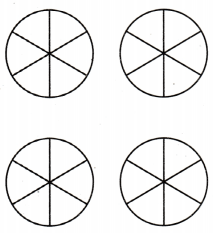The difference is $$\frac{1}{6}$$.
So, there are __________ quesadillas left.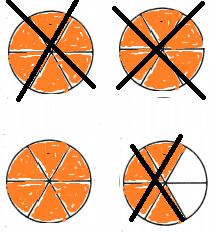The difference is _________ .
So, there are 1$$\frac{1}{6}$$.quesadillas left.
Explanation:
$$\frac{4}{6}$$. – $$\frac{3}{6}$$.
$$\frac{1}{6}$$.
Then subtract the wholes
3 – 2 = 1 Converted $$\frac{1}{6}$$. to 1$$\frac{1}{6}$$.

Record

Subtract the fractional parts of the mixed numbers.
Then subtract the whole-number parts of the mixed numbers.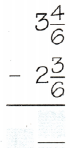Explanation:
Subtracted the fractions first
$$\frac{4}{6}$$. – $$\frac{3}{6}$$.
$$\frac{1}{6}$$.
Then subtract the wholes
3 – 2 = 1 Converted $$\frac{1}{6}$$. to 1$$\frac{1}{6}$$.

Share and Grow

Write the sum as a mixed number with the fractional part less than 1.

Question 1.Explanation:
written the sum

Question 2.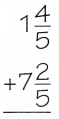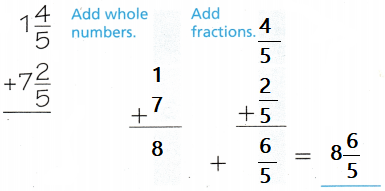Explanation:
written the sum

Question 3.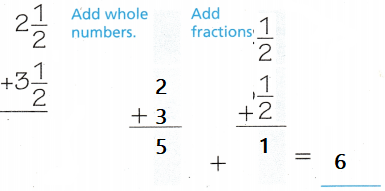Explanation:
written the sum

Find the difference.

Question 4.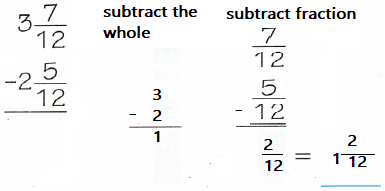Explanation:
subtracted the wholes then subtracted the fractions
written the Difference

Question 5.Explanation:
subtracted the wholes then subtracted the fractions
written the Difference

Question 6.Explanation:
subtracted the wholes then subtracted the fractions
written the Difference

Math Talk

Mathematical Processes
Explain how adding and subtracting mixed numbers is different from adding and Subtracting fractions.
In fractions first we make denominators equal
then add or subtract the fractions directly
Explanation:
In mixed fractions first add or subtract the wholes
then add or subtract the fractions

Problem Solving

Question 7.
The driving distance from Alex’s house to the museum is 6$$\frac{7}{10}$$ miles. What is the round-trip distance?
Answer: 13$$\frac{4}{10}$$ is the round trip distance
Explanation:
6$$\frac{7}{10}$$ + 6$$\frac{7}{10}$$
$$\frac{7}{10}$$ + $$\frac{7}{10}$$  = $$\frac{14}{10}$$
6 + 6 = 12
6 + 6 + 1 + $$\frac{4}{10}$$ = 13$$\frac{4}{10}$$

Question 8.
H.O.T. Apply Multi-Step The driving distance from the sports arena to Kristina’s house is 10$$\frac{9}{10}$$ miles. The distance from the sports arena to Luke’s house is 2$$\frac{7}{10}$$ miles. How much greater is the driving distance between the sports arena and Kristina’s house than between the sports arena and Luke’s house?
Answer: 8$$\frac{2}{10}$$
Explanation:
10$$\frac{9}{10}$$ – 2$$\frac{7}{10}$$ miles.
Subtract the wholes 10 – 2 = 8
then subtract the fractions $$\frac{9}{10}$$ – $$\frac{7}{10}$$
8$$\frac{2}{10}$$ is the driving distance between the sports arena and Kristina’s house than between the sports arena and Luke’s house

Question 9.
Benji biked from his house to the nature preserve, a distance of 23$$\frac{4}{5}$$ miles. Jade hiked from her house to the lake, a distance of 12$$\frac{2}{5}$$ miles. How many fewer miles did Jade bike than Benji?
Answer: 11$$\frac{2}{5}$$ fewer miles did Jade bike than Benji
Explanation:
23$$\frac{4}{5}$$ – 12$$\frac{2}{5}$$
Subtract the wholes 23 – 12 = 11
then subtract the fractions $$\frac{4}{5}$$  – $$\frac{2}{5}$$ = $$\frac{2}{5}$$
11$$\frac{2}{5}$$

Question 10.
H.O.T. Apply During the Samson family trip, they drove from home to a ski lodge, a distance of 55$$\frac{4}{5}$$ miles, and then drove an additional 12$$\frac{4}{5}$$ miles to visit friends. If the family drove the same route back home, what was the distance traveled during their trip?
Answer: 68$$\frac{3}{5}$$
55$$\frac{4}{5}$$ + 12$$\frac{4}{5}$$
add the wholes 55 + 12 = 67
$$\frac{4}{5}$$ + $$\frac{4}{5}$$ = $$\frac{8}{5}$$ = 1$$\frac{3}{5}$$
68$$\frac{3}{5}$$

Question 11.
A chameleon’s body is 1$$\frac{4}{6}$$ feet long. Its tongue is 2$$\frac{5}{6}$$ feet long. How much longer is the chameleon’s tongue than its body?
(A) 2$$\frac{3}{6}$$
(B) 1$$\frac{3}{6}$$
(C) 1$$\frac{1}{6}$$
(D) 2$$\frac{1}{6}$$
Explanation:
2$$\frac{5}{6}$$ – 1$$\frac{4}{6}$$  = 1$$\frac{1}{6}$$ longer is the chameleon’s tongue than its body

Question 12.
Jill rides her horse 5$$\frac{6}{12}$$ miles on a horse trail. She will ride 4$$\frac{5}{12}$$ miles more to reach the end of the trail. How long is the horse trail?
(A) 1$$\frac{1}{12}$$
(B) 1$$\frac{11}{12}$$
(C) 9$$\frac{1}{12}$$
(D) 9$$\frac{11}{12}$$
Explanation:
5$$\frac{6}{12}$$ + 4$$\frac{5}{12}$$ = 9$$\frac{11}{12}$$ is the horse trail

Question 13.
Multi-Step Students bring 8$$\frac{7}{8}$$ gallons of lemonade to a picnic. They drink 5$$\frac{2}{8}$$ gallons with lunch. Then they drink 2$$\frac{1}{8}$$ gallons with an afternoon snack. How much lemonade is left?
(A) 3$$\frac{5}{8}$$ gallons
(B) 6$$\frac{3}{4}$$gallons
(C) 5$$\frac{3}{4}$$gallons
(D) 1$$\frac{1}{2}$$gallons
Explanation:
5$$\frac{2}{8}$$ + 2$$\frac{1}{8}$$ = 7$$\frac{3}{8}$$
8$$\frac{7}{8}$$ – 7$$\frac{3}{8}$$ = 1$$\frac{4}{8}$$
= 1$$\frac{1}{2}$$gallons lemonade is left

TEXAS Test Prep

Question 14.
Jeff used 4$$\frac{7}{8}$$ cups of orange juice and 3$$\frac{1}{8}$$ cups of pineapple juice to make a tropical punch. How much more orange juice than pineapple juice did Jeff use?
(A) $$\frac{3}{4}$$
(B) 1$$\frac{3}{4}$$
(C) 1$$\frac{7}{8}$$
(D) 8 cups
Explanation:
4$$\frac{7}{8}$$ – 3$$\frac{1}{8}$$ = 1$$\frac{6}{8}$$
1$$\frac{3}{4}$$ more orange juice than pineapple juice that Jeff use

### Texas Go Math Grade 4 Lesson 5.6 Homework and Practice Answer Key

Write the sum as a mixed number with the fractional part less than 1.

Question 1.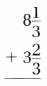Explanation:
written the sum

Question 2.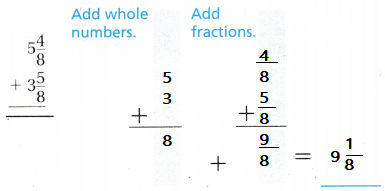Explanation:
written the sum

Question 3.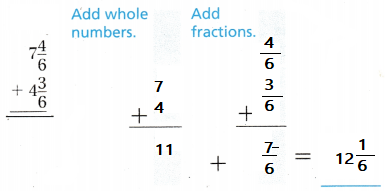Explanation:
written the sum

Question 4.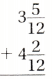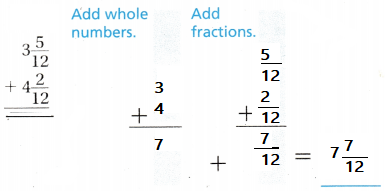Explanation:
written the sum

Find the difference

Question 5.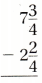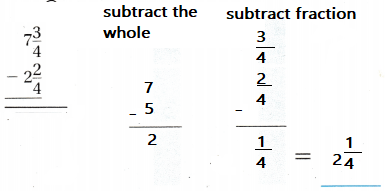Explanation:
subtracted the wholes then subtracted the fractions
written the Difference

Question 6.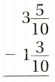Explanation:
subtracted the wholes then subtracted the fractions
written the Difference

Question 7.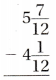Explanation:
subtracted the wholes then subtracted the fractions
written the Difference

Question 8.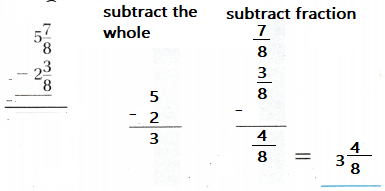Explanation:
subtracted the wholes then subtracted the fractions
written the Difference

Problem Solving

Question 9.
Mrs. Baker drove 2$$\frac{4}{10}$$ hours to visit her mother. It took her 3$$\frac{6}{10}$$ hours to get home. How much longer did it take Mrs. Baker to get home?
Answer: 1$$\frac{2}{10}$$
Explanation:
3$$\frac{6}{10}$$ – 2$$\frac{4}{10}$$  = 1$$\frac{2}{10}$$
1$$\frac{2}{10}$$ longer that take Mrs. Baker to get home

Question 10.
Monica’s recipe calls for 2$$\frac{3}{4}$$ cup of water and 3$$\frac{3}{4}$$ cup of milk. What is the total amount of liquid in the recipe?
Answer:  6$$\frac{2}{4}$$
2$$\frac{3}{4}$$ + 3$$\frac{3}{4}$$ = 6$$\frac{2}{4}$$
6$$\frac{2}{4}$$ is the total amount of liquid in the recipe

Lesson check

Question 11.
Kimberly’s kite tail is 5$$\frac{5}{6}$$ feet long. Margaret’s kite tail is 4$$\frac{3}{6}$$ feet long. How much longer is Kimberly’s kite tail than Margaret’s kite tail?
(A) 1$$\frac{2}{3}$$feet
(B) 1$$\frac{1}{3}$$feet
(C) 2$$\frac{1}{3}$$feet
(D) 2$$\frac{2}{3}$$feet
Explanation:
5$$\frac{5}{6}$$ – 4$$\frac{3}{6}$$  = 1$$\frac{2}{6}$$
1$$\frac{1}{3}$$feet  longer is Kimberly’s kite tail than Margaret’s kite tail.

Question 12.
Wayne recorded his exercise for two months. He walked 2$$\frac{8}{10}$$ miles the first day. He walked 1$$\frac{5}{10}$$ miles the second day. What is the total distance he walked during the two days?
(A) 4$$\frac{3}{10}$$ miles
(B) 4$$\frac{2}{10}$$ miles
(C) 3$$\frac{3}{10}$$ miles
(D) 3$$\frac{2}{10}$$ miles
Explanation:
2$$\frac{8}{10}$$ + 1$$\frac{5}{10}$$ = 3$$\frac{13}{10}$$ = 4$$\frac{3}{10}$$ miles is the total distance he walked during the two days

Question 13.
Kris used 7$$\frac{5}{12}$$ inches of tape to wrap her brother’s gift and 6$$\frac{9}{12}$$ inches of tape to wrap her sister’s gift. What is the total amount of tape Kris used to wrap the gifts?
(A) 13$$\frac{1}{6}$$ inches
(B) 14$$\frac{1}{6}$$ inches
(C) 13$$\frac{1}{12}$$ inches
(D) 14$$\frac{1}{12}$$ inches
Explanation:
7$$\frac{5}{12}$$ + 6$$\frac{9}{12}$$  = 13$$\frac{14}{12}$$ = 14$$\frac{2}{12}$$ is the total amount of tape Kris used to wrap the gifts

Question 14.
The mall is 6$$\frac{6}{10}$$ miles from Miranda’s house. The nearest grocery store is 4$$\frac{2}{10}$$ miles from her house. How much farther is the mall than the grocery store from Miranda’s house?
(A) 2$$\frac{2}{5}$$ miles
(B) 4$$\frac{4}{5}$$ mi1es
(C) 2$$\frac{2}{5}$$ miles
(D) 8$$\frac{2}{5}$$ miles
Explanation:
6$$\frac{6}{10}$$ – 4$$\frac{2}{10}$$ = 2$$\frac{4}{10}$$ = 2$$\frac{2}{5}$$ miles
2$$\frac{2}{5}$$ miles farther is the mall than the grocery store from Miranda’s house

Question 15.
Multi-Step A tank has 5$$\frac{3}{4}$$ gallons of water in it. Today, 4$$\frac{1}{4}$$ gallons of the water is used. Then, the tank is filled with another 6$$\frac{3}{4}$$ gallons of water. What is the amount of water in the tank now?
(A) 8$$\frac{1}{4}$$ gallons
(B) 1$$\frac{1}{2}$$ gallons
(C) 8$$\frac{1}{4}$$ gal1ons
(D) 1$$\frac{3}{4}$$ gallons
5$$\frac{3}{4}$$ – 4$$\frac{1}{4}$$  = 1$$\frac{2}{4}$$
1$$\frac{2}{4}$$ + 6$$\frac{3}{4}$$  = 7$$\frac{5}{4}$$ = 8$$\frac{1}{4}$$ gallons
8$$\frac{1}{4}$$ gallons is the amount of water in the tank now

Question 16.
Multi-Step For a candy recipe, Karen will need 4$$\frac{3}{8}$$ cups of dark chocolate chips, 5$$\frac{5}{8}$$ cups milk chocolate chips, and 3$$\frac{4}{8}$$ cups white chocolate chips. What is the total amount of chips needed for the candy recipe?
(A) 12$$\frac{1}{2}$$ cups
(B) 12$$\frac{2}{3}$$ cups
(C) 13$$\frac{2}{3}$$ cups
(D) 13$$\frac{1}{2}$$ cups
$$\frac{3}{8}$$ + $$\frac{5}{8}$$ + $$\frac{4}{8}$$  = $$\frac{12}{8}$$
1$$\frac{4}{8}$$
so, the fraction is 13$$\frac{1}{2}$$ cups